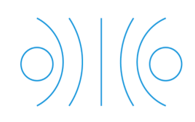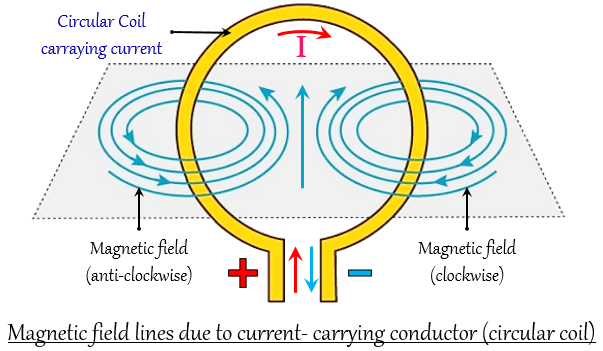(a) a long straight wire  (b) a circular coil  (c) a solenoid  (d) a short straight wire"
">

# The diagram given below represents magnetic field caused by a current-carrying conductor which is:(a) a long straight wire  (b) a circular coil  (c) a solenoid  (d) a short straight wire"

(b) a circular coil

Explanation
The diagram represents a magnetic field caused by a current-carrying conductor which is a circular coil.Updated on: 10-Oct-2022

69 Views